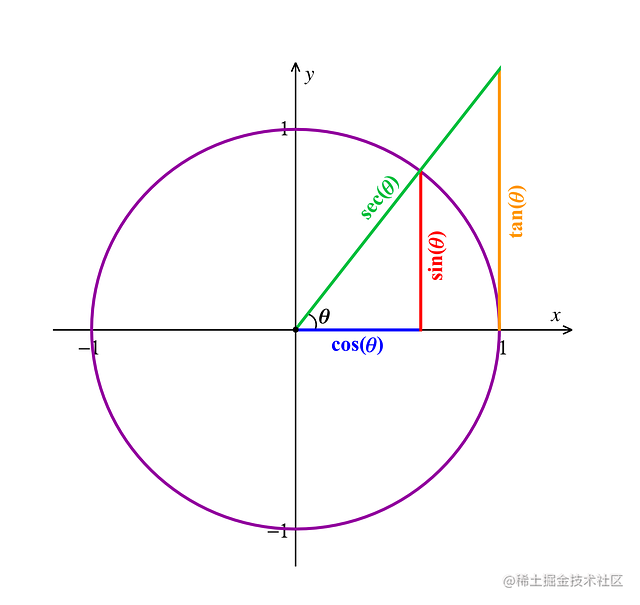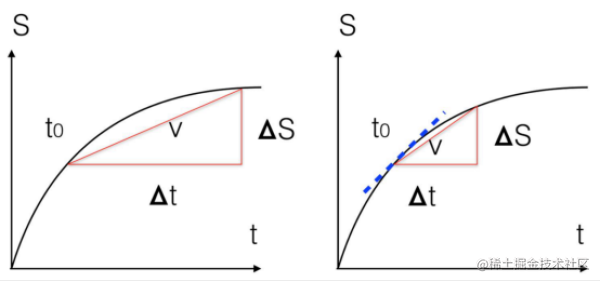# 不懂物理的前端不是好的游戏开发者（一）—— 物理引擎基础### 概述### 代码中的数学

class Vector {
x: number
y: number
z: number
constructor(x: number,y: number,z: number) {
this.x = x
this.y = y
this.z = z
}

setX(x: number) {
this.x = x
}

setY(y: number) {
this.y = y
}

setZ(z: number) {
this.z = z
}
}class VectorOperation {
add (vectorA: Vector, vectorB: Vector) { //  向量相加
return new Vector(
vectorA.x + vectorB.x,
vectorA.y + vectorB.y,
vectorA.z + vectorB.z
)
}

minus (vectorA: Vector, vectorB: Vector) { // 向量相减
return new Vector(
vectorA.x - vectorB.x,
vectorA.y - vectorB.y,
vectorA.z - vectorB.z
)
}

multiply (vectorA: Vector, times: number) { // 向量缩放
return new Vector(
vectorA.x * times,
vectorA.y * times,
vectorA.z * times
)
}

dotProduct (vectorA: Vector, vectorB: Vector) { // 向量点积
return vectorA.x* vectorB.x + vectorA.y* vectorB.y + vectorA.z* vectorB.z
}

vectorProduct (vectorA: Vector, vectorB: Vector) { // 向量外积
return new Vector(
vectorA.y * vectorB.z - vectorA.z * vectorB.y,
vectorA.z * vectorB.x - vectorA.x * vectorB.z,
vectorA.x * vectorB.y - vectorA.y * vectorB.x,
)
}
}

$v = \frac {s_{1} - s_{0}}{t_{1} - t_{0}}$ (平均速度)

$v = \lim_{\Delta t \to 0} \frac {\Delta s}{\Delta t} = \frac{ds}{dt}$ (速度)$s_{1} = s_{0} + v_{0}t$ (匀速运动)

$s_{1} = s_{0} + \int_{t_{0}}^{t_{1}}v_{0}dt$ (匀速运动)

### 物理基础

class GameObj {
pos: Vector
velocity: Vector
acceleration: Vector

constructor(pos?: Vector, velocity?: Vector, acceleration?: Vector) {
this.pos = pos || new Vector(0, 0, 0)
this.velocity = velocity || new Vector(0, 0, 0)
this.acceleration = acceleration || new Vector(0, 0, 0)
}

setPos (pos: Vector) {
this.pos = pos
}

setVelocity (velocity: Vector) {
this.velocity = velocity
}

setAcceleration (acceleration: Vector) {
this.acceleration = acceleration
}
}

$\vec F = m\vec a$ (牛顿第二定律)

class GameObj {
mess: number // 质量不得为 0

constructor(mess?: number) {
if (mess > 0) {
this.mess = mess
}
}

setMess (mess: number) {
if (mess > 0) {
this.mess = mess
}
}
}

class GameObj {
inverseMess: number // 质量不得为 0

constructor(inverseMess?: number) {
if (inverseMess >= 0) {
this.inverseMess = inverseMess
}
}

setInverseMess (inverseMess: number) {
if (inverseMess >= 0) {
this.inverseMess = inverseMess
}
}
}